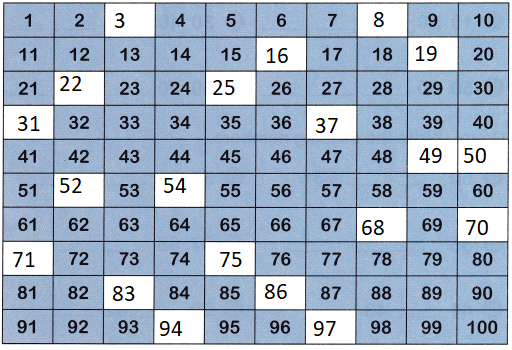All the solutions provided in McGraw Hill Math Grade 1 Answer Key PDF Chapter 5 Test as per the latest syllabus guidelines.

Question 1.
Complete the chart Write the missing numbers.Explanation:
The given numbers are from 1 to 100
some numbers are missing in the given series.

Count on. Write the missing numbers.

Question 2.
53 54 ___ ___ ___
53 54 55 56 57
Explanation:
Some numbers are missing in the given series,
To find the after number we have to add one number
54 + 1 = 55
55 + 1 = 56

Question 3.
60 61 ___ ___ ___
60 61 62 63 64
Explanation:
Some numbers are missing in the given series,
To find the after number we have to add one number
62 + 1 = 63
63 + 1 = 64

Question 4.
56 57 ___ ___ ___
56 57 58 59 60
Explanation:
Some numbers are missing in the given series,
To find the after number we have to add one number

Question 5.
67 70 ___ ___ ___
67 70 73 76 79
Explanation:
Some numbers are missing in the given series,
To find the after number we have to add 3 number
67 + 3 = 70
70 + 3 = 73
73 + 6 = 76

Question 6.
71 72 ___ ___ ___
71 72 73 74 75
Explanation:
Some numbers are missing in the given series,
To find the after number we have to add one number

Question 7.
79 80 ___ ___ ___
79 80 81 82 83
Explanation:
Some numbers are missing in the given series,
To find the after number we have to add one number

Question 8.
96 97 ___ ___ ___
96 97 98 99 100
Explanation:
Some numbers are missing in the given series,
To find the after number we have to add one number

Question 9.
77 78 ___ ___ ___
77 78 79 80 81
Explanation:
Some numbers are missing in the given series,
To find the after number we have to add one number

Question 10.
87 88 ___ ___ ___
87 88 89 90 91
Explanation:
Some numbers are missing in the given series,
To find the after number we have to add one number

Question 11.
89 90 ___ ___ ___
89 90 91 92 93
Explanation:
Some numbers are missing in the given series,
To find the after number we have to add one number

Count by 10s. Write the missing numbers.

Question 12.
10 20 ___ ___ ___
10 20 30 40 50
Explanation:
The given series is skip by tens
Some numbers are missing in the given series,
To find the after number we have to add tens

Question 13.
50 60 ___ ___ ___
50 60 70 80 90
The given series is skip by tens
Explanation:
Some numbers are missing in the given series,
To find the after number we have to add tens

Question 14.
30 40 ___ ___ ___
30 40 50 60 70
Explanation:
The given series is skip by tens
Some numbers are missing in the given series,
To find the after number we have to add tens

Question 15.
60 70 ___ ___ ___
60 70 80 90 100
Explanation:
The given series is skip by tens
Some numbers are missing in the given series,
To find the after number we have to add tens

Count. Write the missing numbers.

Question 16.
50 __ ___ 53
50 51 52 53
Explanation:
Some numbers are missing in the given series,
To find the after number we have to add one number

Question 17.
85 ___ ___ ___
85 86 87 88
Explanation:
Some numbers are missing in the given series,
To find the after number we have to add one number

Question 18.
41 ___ 43 ___
41 42 43 44
Explanation:
Some numbers are missing in the given series,
To find the after number we have to add one number

Question 19.
39 40 ___ ___
39 40 41 42
Explanation:
Some numbers are missing in the given series,
To find the after number we have to add one number

Question 20.
96 97 ___ ___
96 97 98 99
Explanation:
Some numbers are missing in the given series,
To find the after number we have to add one number

Question 21.
33 34 ___ 36 ___
33 34 35 36 37
Explanation:
Some numbers are missing in the given series,
To find the after number we have to add one number

Question 22.
67 68 ___ ____
67 68 69 70 71
Explanation:
Some numbers are missing in the given series,
To find the after number we have to add one number

Question 23.
80 ___ 82 ___
80 81 82 83
Explanation:
Some numbers are missing in the given series,
To find the after number we have to add one number

Question 24.
91 ___ ___ ___
91 92 93 94
Explanation:
Some numbers are missing in the given series,
To find the after number we have to add one number

Question 25.
17 ___ ___ 20